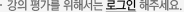### 주메뉴

### 신호 및 시스템

• 부산대학교
• 김형남
• 주제분류
공학 >전기ㆍ전자 >전자공학
• 강의학기
2013년 2학기
• 조회수
15,363
•
1. 강의목표

본 교과목은 전자, 전기 및 컴퓨터 분야를 전공하는 학생들을 대상으로 통신, 제어, 디지털 신호처리 분야 등에서 다루어지는 신호에 대한 개념을 이해하고 아날로그 및 디지털 시스템에서 이를 효과적으로 사용하기 위한 표현 방법 및 해석 방법을 배운다.

2. 강의내용

아날로그 및 디지털 시스템을 다루기 위한 선수 과목으로서 신호의 sampling, 변조(modulation)와 여과(filtering)을 취급하기 위하여 연속시간 시스템과 이산 시간 시스템의 입력 출력 신호들의 관계를 공부하며, Fourier 변환, Z-변환, Discrete-time Fourier 변환 등을 배운다.

3. 교수방법

강의를 통해 이론적인 지식을 습득하도록 하며, 연습문제 풀이 및 MATLAB 등을 이용한 컴퓨터 연습 과제를 통해 이론적인 지식에 대한 이해도 및 적응성을 높일 수 있도록 한다.

#### 차시별 강의1신호및시스템 개요 I - 신호의 에너지와 파워 - 독립 변수의 변환 - Exponential and sinusoidal signal2신호및시스템 개요 II Harmonically related complex exponentials, Sinusoidal & Exponential signals, Unit impulse and Unit step function3선형 시불변 시스템 I Convolution Sum, Convolutional Integral4선형 시불변 시스템 II Properties of LTI systems, Causal LTI systems described by differential and difference equations5주기신호의 푸리에 급수 표현 I The response of LTI systems to complex exponentials, Fourier Series Representation of continuous-time periodice signals, Convergence of the Fourier series6주기 신호의 푸리에 급수 표현 II Properties of continuous-time Fourier series, Fourier series representation of discrete-time periodic signals, Properties of discrete-time Fourier series, Fourier series and LTI systems, Filtering, Examples of continuous-time filters described by differential equations, Examples of discrete-time filters described by difference equations7연속 시간 푸리에 변환 I Representation of aperiodic signals : the continuous-time Fourier transform, The Fourier transform for periodic signals,8연속 시간 푸리에 변환 II Properties of the continuous-time Fourier transform, Systems characterized by linear constant-coefficient differential equations9이산 시간 푸리에 변환 I Representation of Aperiodic Signals : The Discrete-time Fourier Transform, The Fourier transform for periodic signals, Properties of the discrete-time Fourier transform10이산 시간 푸리에 변환 II Properties of discrete-time Fourier transform, Systems characterized by linear constant-coefficient difference equation11샘플링 I The sampling theorem, Reconstruction of a signal from its samples using interpolation, The effect of undersampling, Discrete-time processing of continuous-time signals12샘플링 II Conversion of a discrete-time sequence to a continuous-time signal, SAMPLING OF DISCRETE-TIME SIGNALS13z-변환 I The z-transform, The Region of Convergence for the z-Transform, The Inverse z-Transform, Geometric Evaluation of the Fourier Transform from the Pole-Zero Plot14z-변환 II Properties of the z-Transform, Analysis and Characterization of LTI Systems using z-Transforms, System function algebra and block diagram representations, The Unilateral z-Transform#### 연관 자료#### 사용자 의견#### 이용방법

• 문서 자료 이용시 필요한 프로그램 [바로가기]

※ 강의별로 교수님의 사정에 따라 전체 차시 중 일부 차시만 공개되는 경우가 있으니 양해 부탁드립니다.